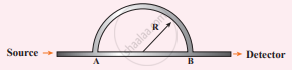Tamil Nadu Board of Secondary EducationHSC Science Class 11th

# A sound wave is transmitted into a tube as shown in the figure. The sound wave splits into two waves at point A which recombine at point B. - Physics

Numerical

A sound wave is transmitted into a tube as shown in the figure. The sound wave splits into two waves at point A which recombine at point B. Let R be the radius of the semi-circle which is varied until the first minimum. Calculate the radius of the semi-circle if the wavelength of the sound is 50.0 m.#### Solution

Given: λ = 50m

To find: R = ??

Path difference = πR – 2R

[Here AB = 2R; Semicircle path = πR]

Formula:

Path difference = (2n – 1)$\frac{λ}{2}$ (for minimum n -1)

R(π - 2) = $\frac{\lambda}{2}$

∴ R = lambda/(2(pi - 2))

= 50/(2[22/7 - 2])

= 50/(2[(22- 14)/7])

= (50 xx 7)/(2 xx 8)

= 21.875

= 21.9 m

Concept: Standing Waves
Is there an error in this question or solution?

Share